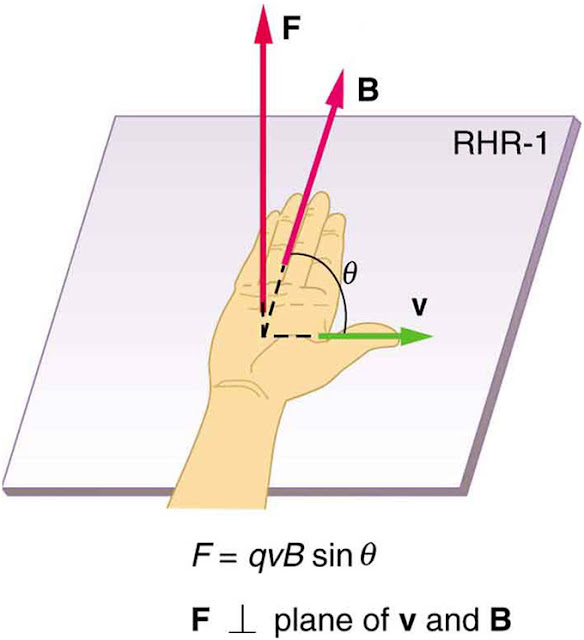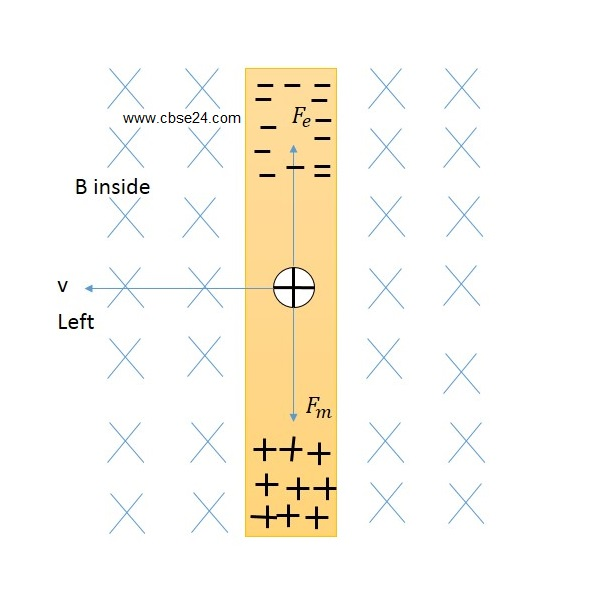# Motional Emf From Lorentz Force | cbse24.com

### Lorentz Force:

In physics(specifically in electromagnetism) the Lorentz force(or electromagnetic force) is the combination of the electric and magnetic force on a point charge due to electromagnetic fields. A particle of charge q moving with a velocity v in an electric field E and a magnetic field B experiences a force of:.

F=qE+qvB

### Motional Emf From Lorentz Force:

A conductor has a large number of free electrons. When it moves through a magnetic field, a Lorentz force acting on the free electrons can set up a current. A rectangular conductor in which arm PQ is free to move. It is placed in a uniform magnetic field B, directed normally into the plane of the paper. As the arm, PQ is moved towards left with speed v,Motional Emf from Lorentz force

the free electron of PQ also moves with the same speed towards left. The electron experience a magnetic Lorentz force Fm=qvB

Lorentz Force is perpendicular to B and v.

According to Right-Hand Palm Rule, this force acts in the direction of PQ. Lorentz Force act at downside if the conductor's arm moves on the left side and Lorentz force will be on the upside if the conductor's arm moves on the right side according to Right Hand Plam Rule. You can see by this picture of right-hand plam rule.Right Hand Plam Rule

Hence the free electrons will move towards PQ. A negative charge accumulates at P and a positive charge at Q.An An electric field E is set up in the conductor from Q to P.This field exerts a force Fe=qE  on the free electrons.

The accumulation of charges at the two ends continues till these two forces balance each otherLorentz force  when the conductor moves on the left side

If the Conductor's arm is moving on the right side than Lorentz force and electric force should be like this manner.....Lorentz force when a conductor moves in the right side

Fm=Fe

qvB=qE

vB=E

The potential difference between the ends Q and P is

V=E.l=vBl

Clearly, it is the magnetic force on the moving free electrons that maintains the potential difference and produces the emf

e=Blv

This emf is produced due to the motion of a conductor, so it is called a motional emf.

Current induced in the loop:
Let R be the resistance of the movable arm PQ of the rectangular loop PQRS in the figure the total resistance of the remaining arms QR, RS and SP is negligible compared to R.Then the current in the loop will be

I=eR=BlvR

Force on the movable arm:
The conductor PQ of length l and carrying current I experiences force F in the perpendicular magnetic field B.

The force is given by

F=IBlsin90=BlvR=B2l2vR

This force (due to induced current) acts downwards direction for left side conductor arm moves.

Power delivered by the external force:
The power supplied by the external force to maintain the motion of the movable arm is

P=Fv=B2l2v2R

The power dissipated as joule loss:
The power dissipated in the loop as Joule heating loss is

P=I2R=(BlvR)2R=B2l2v2RThe equivalent electrical circuit of the electromagnetic setup

Clearly, the mechanical energy expended to maintain the motion of the movable arm is first converted into electrical energy(te induced emf) and then to thermal energy. This is consistent with the law of conservation of energy. This fact allows us to represent the electromagnetic setup.

F=qE+qvB

*